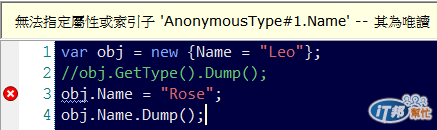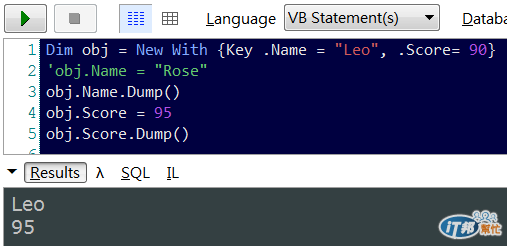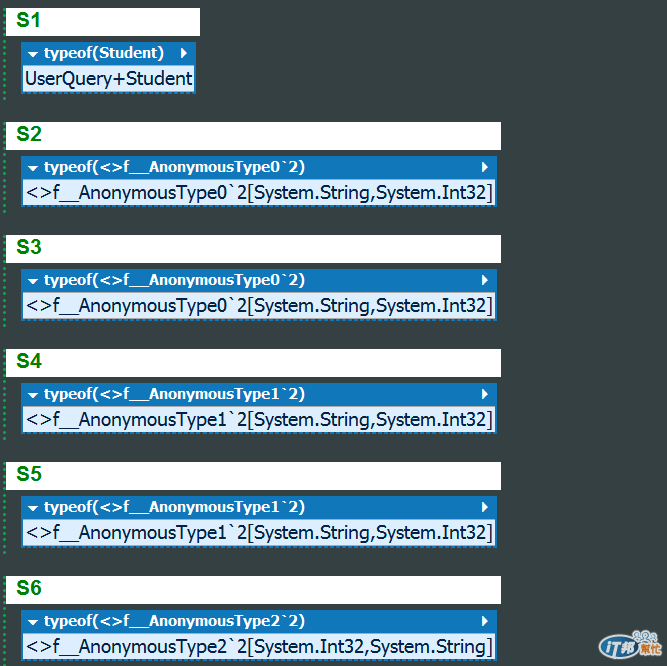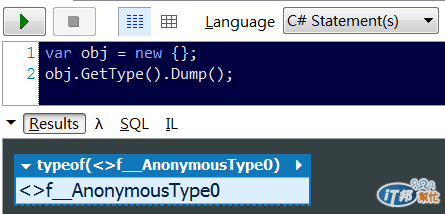#DAY 12
3

## LINQ自學筆記-打地基-匿名型別

MSDN 中，對於 C# 匿名型別的定義如下：

C#：

``````var a = new { Name = "Leo", Score = 90 };
``````

VB：

``````Dim product = New With {.Name = "Leo", .Score= 90}
``````

``````var obj = new {Name = "Leo"};
obj.Name = "Rose";
obj.Name.Dump();
````````````Dim obj = New With {Key .Name = "Leo", .Score= 90}
'obj.Name = "Rose"
obj.Name.Dump()
obj.Score = 95
obj.Score.Dump()
``````1. 屬性數量相同。
2. 屬性指定順序相同。
3. 屬性的型別相同。
4. 屬性的名稱相同。

``````void Main()
{
var s1 = new Student(){StudentName = "Leo", Score = 90};
var s2 = new {StudentName = "Leo", Score = 90};
var s3 = new {s1.StudentName, s1.Score};
s1.GetType().Dump("S1");
s2.GetType().Dump("S2");
s3.GetType().Dump("S3");

var s4 = new {Name = "Rose", Score = 60};
var s5 = new {s4.Name, s4.Score};
var s6 = new {s4.Score, s4.Name};
s4.GetType().Dump("S4");
s5.GetType().Dump("S5");
s6.GetType().Dump("S6");
}

public class Student
{
public string StudentName {get; set;}
public int Score {get; set;}
}
``````